Courses

# Test: Electrochemistry - 3

## 30 Questions MCQ Test Physical Chemistry | Test: Electrochemistry - 3

Description
This mock test of Test: Electrochemistry - 3 for Chemistry helps you for every Chemistry entrance exam. This contains 30 Multiple Choice Questions for Chemistry Test: Electrochemistry - 3 (mcq) to study with solutions a complete question bank. The solved questions answers in this Test: Electrochemistry - 3 quiz give you a good mix of easy questions and tough questions. Chemistry students definitely take this Test: Electrochemistry - 3 exercise for a better result in the exam. You can find other Test: Electrochemistry - 3 extra questions, long questions & short questions for Chemistry on EduRev as well by searching above.
QUESTION: 1

Solution:
QUESTION: 2

Solution:
QUESTION: 3

### At infinite dilution state, the solut ion of CH3COOH in water does not contain:

Solution:
QUESTION: 4

The minimum equivalent conductance in fused state is shown by:

Solution:
QUESTION: 5

According to Kohlrausch law, the limiting value of equivalent conductivity of an electrolyte, A2B is given by:

Solution:
QUESTION: 6

The molecular conductivity and equivalent conductivity are same for the solution of:

Solution:
QUESTION: 7

Variation of molar conductivity with concentration of strong electrolyte is given by Huckel-Onsager equation expressed as:

Solution:
QUESTION: 8

The molar conductivity of NaCl, HCl and CH3COONa at infinite dilution are 126.45, 426.16 and 91 S cm2 mol–1 respectively. The molar conductivity of CH3COOH at infinite dilution is:

Solution:
QUESTION: 9

The conductivity of N/10 KCl solution at 20°C is 0.0212 S cm–1 and the resistance of cell containing this solution at 20°C is 55 ohm. The cell constant is:

Solution:
QUESTION: 10

The limiting molar conductivities of NaCl, KBr and KCl are 126, 152 and 150 S cm2 cm–1 respectively. The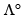for NaBr is:

Solution: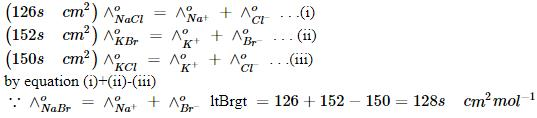QUESTION: 11

The equivalent conductivity o f 0.1 M weak acid is 100 times less than that at infinite dilution. The degree of dissociation of weak electrolyte at 0.1 M is:

Solution:
QUESTION: 12

Limiting molar ionic conductivities of uni-univalent electrolyte are 57 and 73. The limiting molar conductivity of the solution will be:

Solution:
QUESTION: 13

Which statement is not correct for Kohlrausch law:

Solution:
QUESTION: 14

Molar conductivity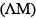and equivalent conductivity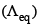for an electrolyte AxBy at any dilution are related as:

Solution:
QUESTION: 15

The charge on cation and anion of an electrolyte is n+ and v- respectively. One molecule of electrolyte furnishes ‘c’ cations and ‘a’ anions. Which expression is not correct:

Solution:
QUESTION: 16

Which statement is correct about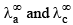(i.e., ionic conductivit ies):

Solution:
QUESTION: 17

Which is correct relation:

Solution:
QUESTION: 18

The conductivity k(in S m–1) is related with molar conductivity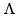(in S m2 mol–1) by the relation (M is molarity in mol m–3)

Solution:
QUESTION: 19

Cell constant of a conductivity cell is generally obtained by measuring conductance of aqueous solution of:

Solution:
QUESTION: 20

The conductivity of 1 × 10–3 M acetic acid is 5 × 10–5 S cm–1 andis 390.5 S cm–1 mol–1. The dissociation constant of acetic acid is:

Solution:
QUESTION: 21

Which of the following shows, the highest electrical conductance in aqueous solution:

Solution:
QUESTION: 22

AgNO3(g) was added to an aqueous KCl solution gradually and the conductivity of the solution was measured. The plot of conductance () versus the volume of AgNO3 is:

Solution:
QUESTION: 23

Resistance of 0.2 M solution of an electrolyte is 50 Ω. The specific conductance of the solution is 1.4 S m–1. The resistance of 0.5 M solution of the same electrolyte is 280 Ω. The molar conductivity of 0.5 M solution of the electrolyte in S m2 mol–1 is:

Solution:
QUESTION: 24

The equivalent conductance of NaCl at concentration C and at infinite dilution are λC and λ∞ respectively. The correct relationship between λC and λ is given is:

Solution:
QUESTION: 25

Resistance of a conductivity cell filled with a solution of an electrolyte of concentration 0.1 M is 100 ohm. The conductivity of this solution is 1.29 S m–1. Resistance of the same cell filled with 0.02 M of the same solution if the electrolyte is 520 ohm. The molar conductivity of 0.02 M solution of electrolyte would be:

Solution:
QUESTION: 26

The mean ionic activity coefficient of 0.0005 mol kg–1 CaCl2 in water at 25°C is:

Solution:

Explanation : log¥ = - 0.509|Z+Z-|√I

I = 0.0015

log¥= -0. 509|2×-1|√0.0015

= - 0.0394

¥ = (10) ^-0.0394

= 0.91

QUESTION: 27

According to the Debye-Huckel limiting law, the mean activity coefficient of 5 × 10–4 mol kg–1 aqueous solution of CaCl2 at 25°C is (the Debye-Huckel constant ‘A’ can be taken to be 0.509)]

Solution:
QUESTION: 28

According to the Debye-Huckel limiting law if the concentration of a dilute aqueous solution of KCl is increased 4-fold, the value of ln γ± (γ± is the molal mean ionic activity coefficient) will:

Solution:
QUESTION: 29

For a 1 molal aqueous NaCl solution, the mean ionic activity coefficient (γ±) and the Debye-Huckel limiting law constant (A) are related as:

Solution:
QUESTION: 30

Specific conductance of 0.01 M KCl solution is x ohm–1 cm–1. When conductivity cell is filled with 0.01 M KCl the conductance observed is y ohm–1. When the same cell is filled with 0.01 M H2SO4, the observed conductance was Z ohm–1 cm–1. Hence specific conductance of 0.01 M H2SO4 is:

Solution: